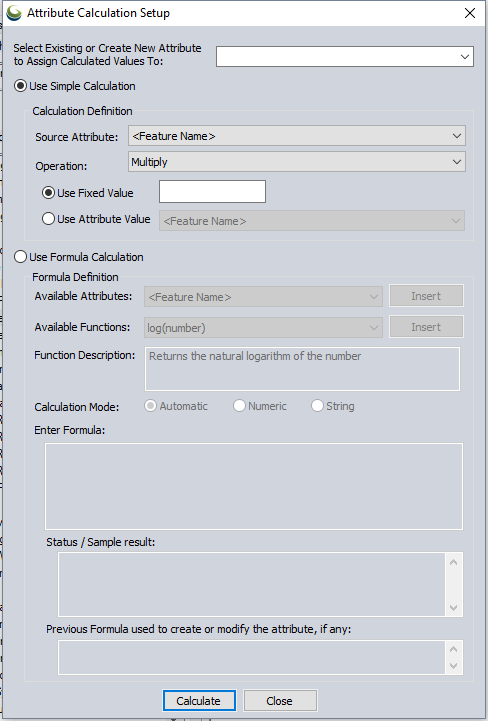#Attribute Calculator

The Attribute Calculator uses the existing values of each feature to updates an existing attribute value or calculate a new attribute value.

Access the Attribute Calculator by pressing theCalculate Attributes button on the Attribute Editor toolbar. The Calculate/ Copy Attribute Values... option is also available from the top level Layer menu, or by Right-click on selected layers in the Control Center and choosing Calculate/ Copy Attribute Values... from the Layer sub-menu.To calculate a new attribute using a simple calculation, perform the following steps:

• At the top of the dialog box the field labeled Select Existing or Create New Attribute to Assign Calculated Values to specifies the output attribute name. Use the drop-down list to update an existing attribute value , or type a new attribute name into the text box to create a new attribute in which to store the calculated value.
• The calculation will be performed on each individual feature based on some existing attribute value of the feature. From the Source Attribute list, choose the starting attribute as the first input value.
• From the Operation list, select the type of mathematical or text operation that will be performed between the input source attribute, and the second input value.
• For the second input, choose between a Fixed Value, and another input Attribute Value.
• Press the Calculate button at the bottom of the dialog to calculate the value and add it to each feature.

## Attribute Name

At the top of the Attribute Calculation Setup dialog, enter the name of the output attribute. This section is labeled Select Existing To update an existing attribute select it from the drop-down list. To create a new attribute type a new attribute name into the text box.

## Use Simple Calculation

The attribute may be calculated by copying the value of another attribute, performing a mathematical operation between two attributes or between an attribute and some number, or by appending a text string to a value when Simple Calculation is selected.

Example

These examples were performed on the default Country data, available by pressing the load default data button on the application start screen.

This simple calculation creates a new attribute called Population Density, which is calculated for each feature taking the POP_EST (population estimate) attribute, and dividing by the AREA attribute.

When the Calculate button is pressed at the bottom of the dialog, a new attribute named Population Density is created, that is calculated from the formula: POP_EST / AREA

In the second example, a new attribute named GDP is added, based on multiplying GDP_MD_EST by 1,000,000. The original attribute represented Gross Domestic Product in millions of dollars, so now the new attribute named GDP contains the dollar amount.

## Use Formula Calculation

Selecting the Use Formula Calculation option enables the use of additional formulas.

The formula is created in the Enter Formula section, but the menus and drop-downs above can be used to help build the formula by inserting the selection into the expression.

To calculate an attribute using a formula, specify the output attribute name at the top of the dialog, and select the Use Formula Calculation to enable this advanced attribute calculation.

• From the Available Functions list, select the desired function. The Function Description box will display an explanation of the selected function. To use a function, press the 'Insert' button to the right of the selected function.
• The text inside of the parenthesis will describe the expected inputs. Highlight the inputs to replace the placeholder descriptions with actual input values. The input values can be typed in, or attributes may be added as inputs using the Available Attributes list and the Insert button.
• Once the formula is set up, check the Status /Sample results section to make sure that the formula is valid, and calculates as expected for the first feature.
• Press the Calculate button at the bottom of the dialog to calculate the attribute value for each feature.

### Available Attributes

Select an attribute to insert into the current formula (use the Insert button to the right)

### Available Functions

User may select a function template to insert into the current formula, which includes the function name and dummy parameters where they're expected. Insert the function using the button immediately to the right. Changing the current function in the list displays a function description in the read-only control directly below the function selector. For a full list of available options see Formula Calculator   The calculator provides a number of formulas to aid in calculation of values. Note that function names are case-insensitive; e.g., abs is the same as ABS

### Enter Formula to Use

This is an editable control to type in the formula that will be processed on each input feature.

Insertions from the Available Attributes and Available Functions drop downs determine the current insertion point in this control, and replace any current selection. As changes are made to the formula, a status is updated in the Status control immediately below.

### Status/ Sample Result

Indicates the status of the current formula. If the formula is non-empty, it is evaluated for correctness. If there is no error, then the status will be 'OK'. Otherwise, if there is an error an indication of what the error is displayed. In addition, if there is no error, then the dialog used the current feature in whatever feature collection is being considered to evaluate the value that the formula would compute, as a sample. This is shown below the status. The parameters (referenced attribute values) are also listed below the sample result.

### Previous Formula

The formula previously used to calculate an attribute will be remembered and can be copied and repasted into the the formula dialog using CTRL+C and CTRL+V keyboard shortcuts. The information displayed here is tied to the attribute listed at the top of the dialog, in Select Existing or Create New Attribute.